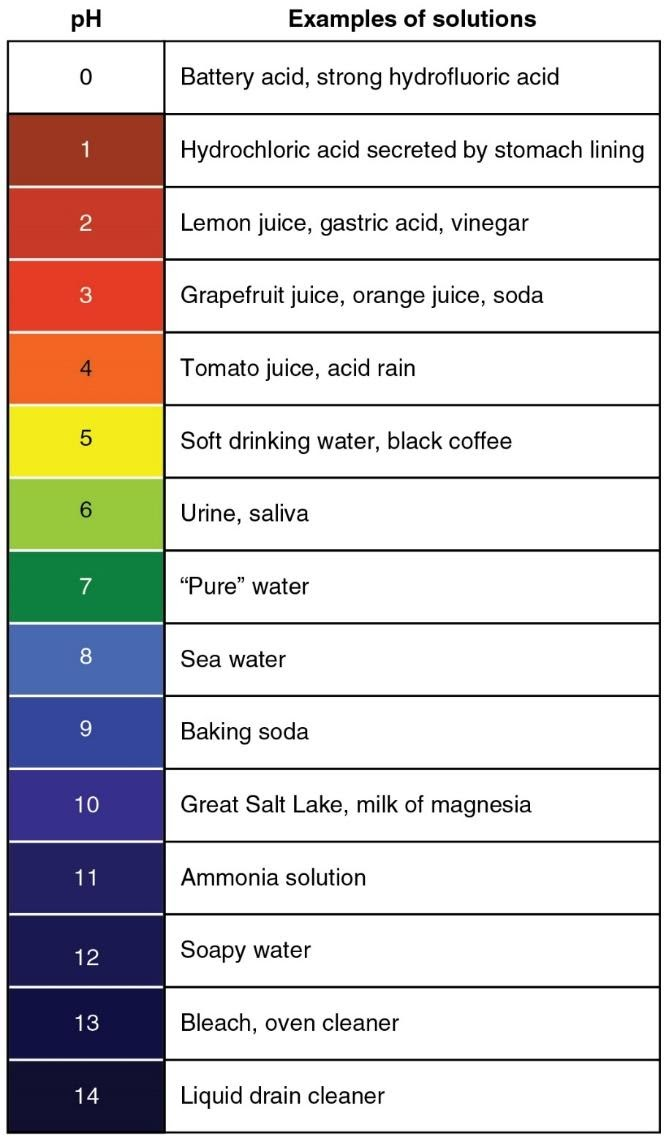2.3.2

# pH

Why do we care about the [H+] anyway? What is special about this particular ion? Well, it turns out that either too much or too little H+ can cause serious problems to the body. If the [H+] is too low, it causes an excitation of the nervous system, resulting in constant contraction of our muscles, including the respiratory muscles, and that is a problem. On the other hand, if the [H+] is too high, it can result in depression of the nervous system, leading to coma. We use the terms acidic and basic to describe these conditions. If the [H+] of the solution is greater than 1.0 x 10-7, we say the solution is acidic, and if the [H+] is less than 1.0 X 10-7, we say the solution is basic.

Because the [H+] is so important and because it is rather cumbersome to say things like, “the [H+] of the fluid is 1.0 X 10-7 Molar,” chemists have developed a shorthand to express the [H+]. This shorthand expresses the [H+] as the pH of the solution. The pH of a solution is the negative logarithm of the [H+] (concentration expressed as moles per liter, M). So, if the [H+] is 1.0 x 10-7 M, the pH of that solution would be 7 (-log 10-7 is -(-7) or 7). Since this is the pH in which the [H+] and [OH-] are equal, we say that this is a neutral solution. When using pH, one thing that is a little confusing is that as the [H+] of a solution goes up, the pH goes down. Suppose that a solution has a [H+] of 1.0 X 10-6 M. The pH of the solution would be 6, but since the math behind pH is log base 10, the change in pH from 7 to a pH of 6 represents a 10 fold increase in hyrdrogen ions. Moving from a pH of 7 to pH of 5 represents a 100 fold increase. Thus, an acidic solution is any solution with a pH<7. Likewise, any solution that has a pH>7 is a basic solution. Below is an image that shows the pH of some common solutions.So, there are two important lessons from this; the lower the pH, the higher the [H+], and a change in pH of one unit (7 to 6 for example) is a 10-fold change in [H+]. Just for reference, the normal pH of our blood is slightly basic, 7.4 (range = 7.35 – 7.45). If the pH of the blood rises above 7.45, the person is in a state of alkalosis (not enough H+), and if it drops below 7.35, the person is in a state of acidosis (too much H+). In mammals, the pH range of the blood that is considered to be compatible with life is from 6.8 to 7.8. A pH above or below these values usually results in death.